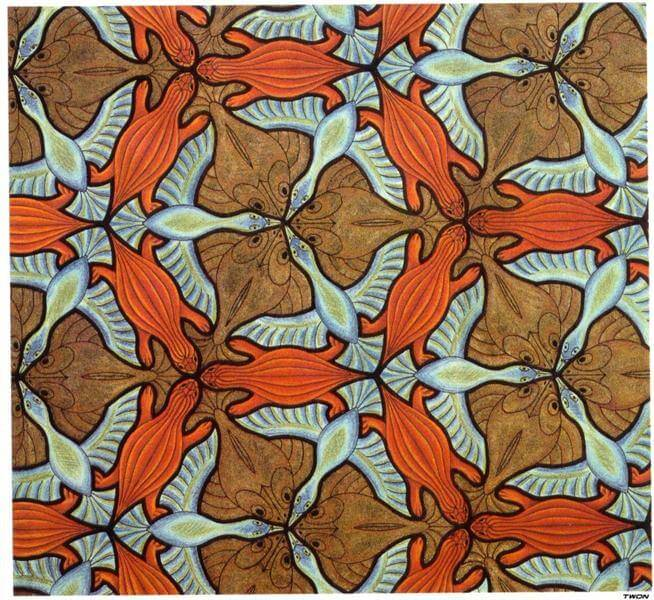# Algorithms: Find the Symmetric Difference in SwiftHey guys Leo here,

Today we will start a series in this blog called: Algorithms, the Final Frontier. We’ll follow the interview prep series from the free code camp.

The first challenge: Challenge Here

The problem: Given two sets (for example set A = {1, 2, 3} and set B = {2, 3, 4}), the mathematical term “symmetric difference” of two sets is the set of elements which are in either of the two sets, but not in both (A △ B = C = {1, 4}).

I could come to this answer:

```var a1 = [1,2,3,4]
var a2 = [5,6,7,8,13]
var a3 = [9,10,11,12,13,13]

func symmetricDiff(arrays: [[Int]]) -> [Int] {

var resultDict : [Int:Int] = [:]

for x in 0..<arrays.count {
let setTemp = Set<Int>(arrays[x])
for value in setTemp {
if resultDict[value] != nil {
resultDict[value] = resultDict[value]! + 1
} else {
resultDict[value] = 1
}
}
}

return Array<Int>(resultDict.filter({ (element) -> Bool in
element.value == 1
}).keys)
}

print(symmetricDiff(arrays: [a1,a2,a3]))
```

OR

A more Swiftly way:

```func symmetricDiffSwiftly(arrays: [[Int]]) -> [Int] {

var finalSet = Set<Int>()
arrays.forEach { array in
finalSet = finalSet.symmetricDifference(array)
}
return Array<Int>(finalSet)
}
```

Pretty straightforward, right?

## Continue Algorithm Studying in Swift

If you like the solution for this challenge, you will love the stack solution for the “matching parenthesis problem”.

Or how about learning the Wirth permutation and solving the combinatory problem called “No Repeats Please”. There you will learn to generate combinations and compare them to check repetition.

Any feedback will be appreciated! Thanks for the reading.

That’s all my people, I hope you liked reading this article as much as I enjoyed writing it. If you want to support this blog you can Buy Me a Coffee or just leave a comment saying hello. You can also sponsor posts and I’m open to `freelance writing!` You can reach me on LinkedIn or Twitter and send me an e-mail through the contact page.

Thanks for the reading and… That’s all folks.

Image Credit: Wikiart